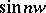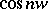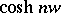# Z-transform

2010 Mathematics Subject Classification: Primary: 05A15 [MSN][ZBL]

Z-transformation

This transform method may be traced back to A. De Moivre [a5] around the year 1730 when he introduced the concept of "generating functions" in probability theory. Closely related to generating functions is the Z-transform, which may be considered as the discrete analogue of the Laplace transform. The Z-transform is widely used in the analysis and design of digital control, and signal processing [a4], [a2], [a3], [a6].

The Z-transform of a sequence $x ( n )$, $n \in \mathbf{Z}$, that is identically zero for negative integers, is defined as

\begin{equation} \tag{a1} \tilde{x} ( z ) = Z ( x ( n ) ) = \sum _ { j = 0 } ^ { \infty } x ( j ) z ^ { - j }, \end{equation}

where $z$ is a complex number.

By the root test, the series (a1) converges if $| z | > R$, where $R = \operatorname { limsup } _ { n \rightarrow \infty } | x ( n ) | ^ { 1 / n }$. The number $R$ is called the radius of convergence of the series (a1).

## Contents

### Example 1.

The Z-transform of $\{ a ^ { n } \}$ is given by

\begin{equation*} Z ( a ^ { n } ) = \sum _ { j = 0 } ^ { \infty } a ^ { j } z ^ { - j } = \frac { z } { z - a } \text { for } | z | > 1. \end{equation*}

### Example 2.

The Z-transform of the Kronecker-delta sequence

\begin{equation*} \delta _ { k } ( n ) = \left\{ \begin{array} { l l } { 1 } & { \text { if } n = k } \\ { 0 } & { \text { if } n \neq k, } \end{array} \right. \end{equation*}

is given by

\begin{equation*} Z ( \delta _ { k } ( n ) ) = \sum _ { j = 0 } ^ { \infty } \delta _ { k } ( j ) z ^ { - j } = z ^ { - k } \text{ for all }z. \end{equation*}

## Properties of the Z-transform.

i) Linearity: Let $R _ { 1 }$ and $R _ { 2 }$ be the radii of convergence of the sequences $x ( n )$ and $y( n )$. Then for any $\alpha , \beta \in \bf{C}$,

\begin{equation*} Z ( \alpha x ( n ) + \beta y ( n ) ) = \alpha Z ( x ( n ) ) + \beta Z ( y ( n ) ), \end{equation*}

\begin{equation*} \text{for} \, | z | > \operatorname { max } \{ R _ { 1 } , R _ { 2 } \}. \end{equation*}

ii) Shifting: Let $R$ be the radius of convergence of $Z ( x ( n ) )$. Then, for $k \in \mathbf Z ^ { + }$,

a) Right-shifting: $Z [ x ( n - k ) ] = z ^ { - k } Z ( x ( n ) )$, for $| z | > R$;

b) Left-shifting: $Z ( x ( n + k ) ) = z ^ { k } Z ( x ( n ) ) - \sum _ { r = 0 } ^ { k - 1 } x ( r ) z ^ { k - r }$, for $| z | > R$.

iii) Initial and final value.

a) Initial value theorem: $\operatorname { lim } _ { |z | \rightarrow \infty } \tilde { x } ( z ) = x ( 0 )$;

b) Final value theorem: $x ( \infty ) = \operatorname { lim } _ { n \rightarrow \infty } x ( n ) = \operatorname { lim } _ { z \rightarrow 1 } ( z - 1 ) Z ( x ( n ) )$.

iv) Convolution: The convolution of two sequences $x ( n )$ and $y( n )$ is defined by

\begin{equation*} x ( n ) ^ { * } y ( n ) = \sum _ { j = 0 } ^ { n } x ( n - j ) y ( j ) = \sum _ { j = 0 } ^ { n } x ( n ) y ( n - j ) \end{equation*}

and its Z-transform is given by

\begin{equation*} Z ( x ( n ) ^ { * } y ( n ) ) = Z ( x ( n ) ) .Z ( y ( n ) ). \end{equation*}

## Inverse Z-transform.

If $\tilde{x} ( z ) = Z ( x ( n ) )$, then the inverse Z-transform is defined as $Z ^ { - 1 } ( \tilde{x} ( z ) ) = x ( n )$. Notice that by Laurent's theorem [a1] (cf. also Laurent series), the inverse Z-transform is unique [a2]. Consider a circle $c$ centred at the origin of the $z$-plane and enclosing all the poles of $z ^ { n - 1 } \tilde{x}(z)$. Then, by the Cauchy integral theorem [a1], the inversion formula is given by

\begin{equation*} x ( n ) = \frac { 1 } { 2 \pi i } \oint _ { c } \widetilde{x} ( z ) z ^ { n - 1 } d z \end{equation*}

and by the residue theorem (cf. also Residue of an analytic function) [a1], $x ( n ) = \sum ( \text { residues of } z ^ { n - 1 } \tilde{x}(z) )$.

If $\tilde{x} ( z ) z ^ { n - 1 } = h ( z ) / g ( z )$ in its reduced form, then the poles of $\tilde{x} ( z ) z ^ { n - 1 }$ are the zeros of $g ( z )$.

a) If $g ( z )$ has simple zeros, then the residue $K_i$ corresponding to the zero $z_i$ is given by

\begin{equation*} K _ { i } = \operatorname { lim } _ { z \rightarrow z _ { i } } \left[ ( z - z _ { i } ) \frac { h ( z ) } { g ( z ) } \right]. \end{equation*}

b) If $g ( z )$ has multiple zeros, then the residue $K_i$ at the zero $z_i$ with multiplicity $r$ is given by

\begin{equation*} K _ { i } = \frac { 1 } { ( r - 1 ) ! } \operatorname { lim } _ { z \rightarrow z _ { i } } \frac { d ^ { n } } { d z ^ { r- 1 } } \left[ ( z - z _ { i } ) ^ { r } \frac { h ( z ) } { g ( z ) } \right] . \end{equation*}

The most practical method of finding the inverse Z-transform is the use of partial-fractions techniques as illustrated by the following example.

### Example.

See also [a2]. Suppose the problem is to solve the difference equation

\begin{equation} x \left( n + 4 \right) + 9 x \left( n + 3 \right) + 30 x \left( n + 2 \right) + 20 x \left( n + 1 \right) + 24 x \left( n \right) = 0 , \end{equation}

where $x ( 0 ) = 0$, $x ( 1 ) = 0$, $x ( 2 ) = 1$, $x ( 3 ) = 10$.

Taking the Z-transform yields

\begin{equation*} Z ( x ( n ) ) = \frac { z ( z - 1 ) } { ( z + 2 ) ^ { 3 } ( z + 3 ) } = \end{equation*}

\begin{equation*} = \frac { - 4 z } { z + 2 } + \frac { 4 z } { ( z + 2 ) ^ { 2 } } - \frac { 3 z } { ( z + 2 ) ^ { 3 } } + \frac { 4 z } { z + 3 }. \end{equation*}

Taking the inverse Z-transform of both sides yields

\begin{equation*} x ( n ) = \left( \frac { 3 } { 4 } n ^ { 2 } - \frac { 11 } { 4 } n - 4 \right) ( - 2 ) ^ { n } + 4 ( - 3 ) ^ { n }. \end{equation*}

## Pairs of Z-transforms.

 $x ( n )$ $Z ( x ( n ) )$ $a ^ { n }$ $z/ z - a$ $n ^ { k }$ $k ! z \,/ ( z - 1 ) ^ { k + 1 }$ $n ^ { k } a ^ { n }$ $( - 1 ) ^ { k } D ^ { k } ( z / ( z - 1 )$; $D = z d / d z$$z\operatorname {sin} w/( z ^ { 2 } - 2 z \operatorname { cos } w + 1 )$$z ( z - \operatorname { cos } w ) / ( z ^ { 2 } - 2 z \operatorname { cos } w + 1 )$ $\delta _ { k } ( n )$ $z ^ { - k }$$z \operatorname{sinh} w/ ( z ^ { 2 } - 2 z \operatorname { cosh } w + 1 )$$z ( z - \operatorname { cosh } w ) / ( z ^ { 2 } - 2 z \operatorname { cosh } w + 1 )$.
How to Cite This Entry:
Z-transform. Encyclopedia of Mathematics. URL: http://encyclopediaofmath.org/index.php?title=Z-transform&oldid=50591
This article was adapted from an original article by S. Elaydi (originator), which appeared in Encyclopedia of Mathematics - ISBN 1402006098. See original article